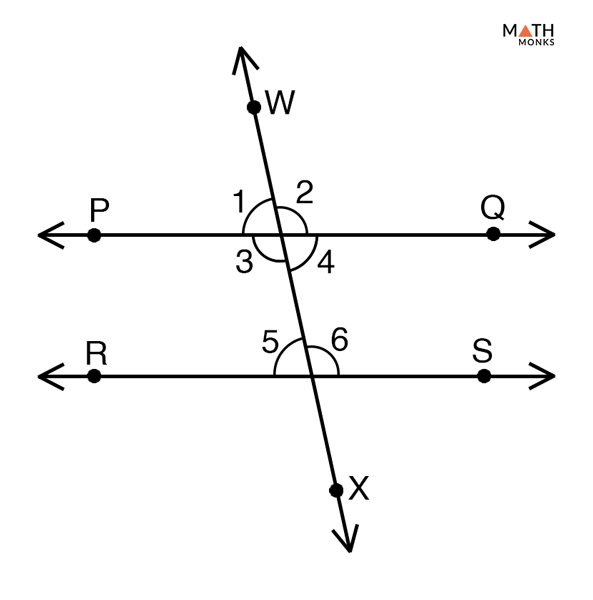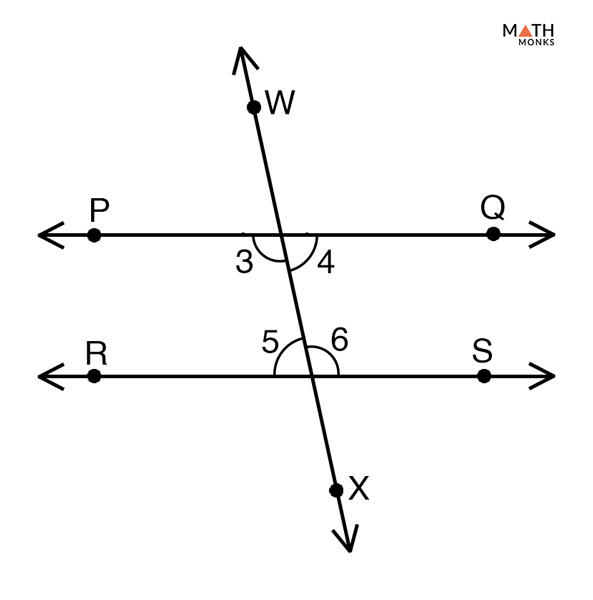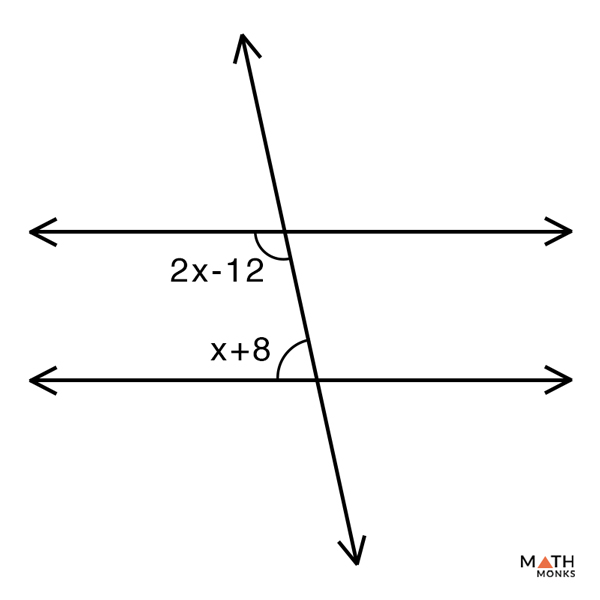# Consecutive Interior Angles

## What are Consecutive Interior Angles

These are pairs of angles that are formed when a transversal line crosses two parallel or non-parallel lines. Consecutive interior angles also known as co-interior angles lie on one side of the transversal but inside of the two lines.

When the two lines are parallel, the pair of consecutive interior angles add up to 180°

The above figure shows two parallel lines AB and CD intersected by the transversal LM. The pair of consecutive interior angles that lie on one side of the transversal but inside of the two lines is given below:

∠1 and ∠2, ∠3 and ∠4

## Consecutive Interior Angles TheoremProve Consecutive Interior Angles Theorem

To prove:

∠3 + ∠5 = 180° and ∠4 + ∠6 = 180°

Proof:

Given that, ‘PQ’ and ‘RS’ are the two parallel lines intersected by the transversal WX
Now, ∠1 = ∠3 (Linear Pair), and
∠2 = ∠4 (Linear Pair)
Then,
∠1 + ∠3 =180° …..(1) (Supplementary Pair)
Similarly,
∠2 + ∠4 =180°……. (2) (Supplementary Pair)
By Corresponding angles theorem,
∠1 =∠5…… (3)
∠2 =∠6……. (4)
Substituting (3) in (1) we get,
∠3 + ∠5 = 180°
Similarly,
Substituting (4) in (2) we get,
∠4 + ∠6 = 180°
Hence Proved

### Converse of Consecutive Interior Angles TheoremProve the Converse of Consecutive Interior Angles Theorem

To prove:

PQ∥ RS

Proof:

Proof: Given that, ∠3 + ∠5 = 180° and ∠4 + ∠6 = 180° are the two pairs of consecutive interior angles that are supplementary
Now, ∠3 + ∠4 = 180° (Supplementary angles)
Thus,
∠3 + ∠4 = ∠4 + ∠6
∠3 = ∠6 (Alternate interior angles)
Thus,
PQ∥ RS
Hence Proved

Let us take some example to understand the concept better,Find the value of x, y and z in the given figure.

Solution:

According to the theorem of consecutive interior angles if the two lines intersected by the transversal are parallel, the consecutive interior angles are supplementary.
Thus,
∠50° + x = 180°
x = 180°- 50°
x = 130°
Now,
y =∠50° (Alternate interior angle)
Similarly,
z = x(Alternate interior angle)
Thus,
z = 130°In the given pair of consecutive interior angles, find the value of x and the magnitude of a consecutive interior angle.

Solution:

According to the theorem of consecutive interior angles the consecutive interior angles are supplementary.
Thus,
2x – 12 = x +8
2x – x = 8 + 12
x = 20
Hence the magnitude,
2x – 12 = 20 x 2 – 12 = 28

## FAQs

Q1. Are consecutive interior angles congruent?

Ans. Consecutive interior angles are not congruent but are supplementary to each other, which means they add up to 180°.